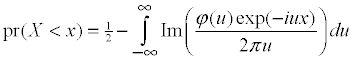Linear combination of chi-squared random variables

X1,...,Xn are independent (non-central) chi-squared random variables and Z is a normal random variable independent of X1,...,Xn. Calculate the probability

Pr(a1 X1 + ... + an Xn + a0Z  <  x).

I wrote a program for doing this and published it in Applied Statistics in 1980. The program was written in Algol so is not much use today. I have translated the program into both Fortran and C. This page documents these programs. You can ftp copies of the source from the download page.

A quadratic form in normal random variables can expressed as a linear combination of chi-squared variables so the method described here can also be used for calculating the distribution of quadratic form in normal random variables.

Fortran version

The Fortran version was originally written in Ratfor and the Fortran presented here is the version produced by the Ratfor translator. So the code looks a little strange.

The source includes a main program. Delete this if you are going to incorporate the qf function into your program. For testing, compile qf.for and run

qf < qf.dat > xxx
and then compare xxx with qf.txt. To run an example with you entering the coefficients just run qf. The data is read in using ordinary Fortran fixed format reads. Integers should be aligned to the right-hand side of their fields, reals including a decimal can be anywhere within their fields. End the session by entering -1 for ir.

Main call:

real function qf (alb, anc, n, irr, sigma, cc, lim1, acc, ith, trace, ifault)
integer irr, lim1, ifault
real sigma, cc, acc
real trace(7), alb(irr), anc(irr)
integer n(irr), ith(irr)

input:

parameter type description
alb real coefficients of chi-squared variables
anc real non-centrality parameters
n integer degrees of freedom
ith integer workspace
irr integer number of coefficients
sigma real coefficient of standard normal variable
cc real point at which cdf is to be evaluated
lim1 integer maximum number of terms in integration (eg 10000)
acc real maximum error (eg 0.0001)

output:

parameter type description
qf real function cdf value
trace real (length 7)
 trace(1) absolute sum trace(2) total number of integration terms trace(3) number of integrations trace(4) integration interval in final integration trace(5) truncation point in initial integration trace(6) s.d. of initial convergence factor trace(7) cycles to locate integration parameters
ifault integer
 0 normal operation 1 required accuracy not achieved 2 round-off error possibly significant 3 invalid parameters

C version

The source includes a main function. Delete this if you are going to incorporate the qf function into your program. For testing, compile and link qfc.c and run
qfc < qf.dat > xxx
and then compare xxx with qfc.txt. To run an example with you entering the coefficients just run qf. The data is read in free format. End the session by entering -1 for r.

The program assumes the floating point variables are of type real. By default real is typedefed to double. You can change this to float if appropriate.

Main call:

real qf(real* lb, real* nc, int* n, int r, real sigma, real c,
int lim, real acc, real* trace, int* ifault)

input:

parameter type description
lb real* coefficients of chi-squared variables
nc real* non-centrality parameters
n int* degrees of freedom
r int number of coefficients
sigma real coefficient of standard normal variable
c real point at which cdf is to be evaluated
lim int maximum number of terms in integration (eg 10000)
acc real maximum error (eg 0.0001)

output:

parameter type description
qf real cdf value
trace real* (length 7)
 trace absolute sum trace total number of integration terms trace number of integrations trace integration interval in final integration trace truncation point in initial integration trace s.d. of initial convergence factor trace cycles to locate integration parameters
ifault int*
 0 normal operation 1 required accuracy not achieved 2 round-off error possibly significant 3 invalid parameters 4 unable to locate integration parameters 5 out of memory

Method

The method is documented in my 1973 and 1980 papers.

I use numerical integration to evaluate the Gil-Pelaez formulausing the simple trapezoidal rule. In the 1973 paper I find an explicit and quite good bound on the integration error.

The 1980 paper derives a variety of bounds on the truncation error, that is the error when the infinite sum required by the trapezoidal rule is replaced by a finite sum. So for a given accuracy, I can work out a numerical integration method which is guaranteed to achieve this accuracy. If the sum of the degrees of freedom of the chi-squared terms is reasonably large, the number of terms required in the integration is usually surprisingly small.

But suppose the linear combination is dominated by a few terms with a low number of degrees of freedom. Then the number of terms required can become excessive. I use two tricks to overcome this. The first by including an integration factor, in effect, by increasing the value of a0. I am able to calculate the error introduced and the procedure is useful unless x is close to zero. The second trick is to evaluate the error introduced by the integration factor by another numerical integration. Again I can find a bound on the error introduced by the numerical integration.

The method works well in most situations if you want only modest accuracy, say 0.0001. But problems may arise if the sum is dominated by one or two terms with a total of only one or two degrees of freedom and  x is small.

Ruben's method is faster if a0 = 0, all the other ai are positive, the non-centralities are not too large and the ratio of the largest to the smallest ai is not too large. There are alternative and possibly faster methods if all the degrees of freedom are even. Other authors have used automatic integration methods with the Gil-Pelaez formulae. The problem with them is you don't really know if these are performing as claimed, particularly for difficult sets of coefficients.